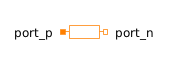Hysteresis - MapleSim Help

Hysteresis

Transform Real to Boolean signal with HysteresisDescription The Hysteresis component uses hysteresis to convert a real input signal to a Boolean output signal.Equations $y=\left({u}_{\mathrm{high}}Connections

 Name Description Modelica ID $u$ Real input signal u $y$ Boolean output signal yParameters

 Name Default Units Description Modelica ID ${u}_{\mathrm{low}}$ $0$ If $y$ is true and $u\le {u}_{\mathrm{low}}$, the output switches to false uLow ${u}_{\mathrm{high}}$ $1$ If y is false and $u\ge {u}_{\mathrm{high}}$, the output switches to true uHigh ${\mathrm{pre}}_{{y}_{0}}$ $\mathrm{false}$ Initial value of the output pre_y_startModelica Standard Library The component described in this topic is from the Modelica Standard Library. To view the original documentation, which includes author and copyright information, click here.